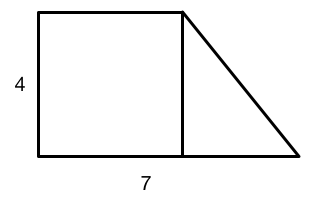# Square + Triangle - Part 2The above figure consists of a square and a triangle. The side lengths of the figure are 4 and 7, as marked.

What is the area of the figure?

×